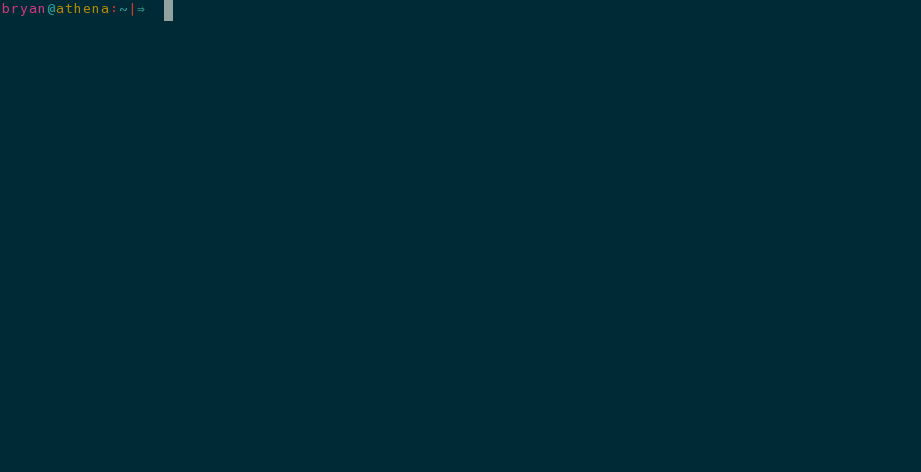# Vimscript Solution for Maintaining Comment Boxes

Even though I know it’s not normally considered a good practice, I still like to use comment boxes from time to time. These are especially handy in bash scripts to write comments about the script at the top.

The reason they are generally considered a bad practice is that they are difficult to change and thus encourage stale documentation. I still advice that you don’t use comment boxes in the real world since not everyone on your team will have read this amazing article. But for small scripts on my own system, I still use comment boxes, and you can too.

## The Code

In the below code snippet, you’ll find a definition for the `MakeBox` vimscript function. This function can be used to help create and maintain comment boxes in any language that has a single line comment specification (e.g. `//` not `/* ... */`).

``````"""""""""""""""""""""""""""""""""""""""""""""""""""""""""""""""""""""""""""""""
" Close comment box.                                                          "
"                                                                             "
" Beginning comment bar and ending comment bar must both be defined already   "
" and the cursor needs to be between the bars when 'MakeBox' is called.       "
"""""""""""""""""""""""""""""""""""""""""""""""""""""""""""""""""""""""""""""""
function! MakeBox()
" Mark starting position
normal! mm

if index(['sh', 'python', 'ruby'], &ft) >= 0
let g:comment_char = '#'
elseif index(['c', 'cpp', 'java', 'javascript', 'php'], &ft) >= 0
let g:comment_char = '/'
elseif index(['haskell', 'sql'], &ft) >= 0
let g:comment_char = '-'
elseif index(['tex'], &ft) >= 0
let g:comment_char = '%'
elseif index(['scheme'], &ft) >= 0
let g:comment_char = ';'
elseif index(['vim'], &ft) >= 0
let g:comment_char = '"'
endif

let triple_comment = g:comment_char . g:comment_char . g:comment_char

let curr_line = getline('.')
while curr_line[0:2] != triple_comment
normal! k
let curr_line = getline('.')
endw

normal! \$
let max_line = col('.')

call MakeBoxBar(max_line)

normal! j
let curr_line = getline('.')
let first_line = 1
while curr_line[0:2] != triple_comment
if first_line == 1
let first_line = 0
else
normal! j
endif

let curr_line = getline('.')
call MakeBoxLine(max_line)
endw

call MakeBoxBar(max_line)

" Return cursor to starting position
normal! `mh
endfunction

"""""""""""""""""""""""""""""""""""""""""""""""""""""""""""""""""""""""""""""""
" Construct one of the bars that goes at the beginning and end of a           "
" comment-box.                                                                "
"""""""""""""""""""""""""""""""""""""""""""""""""""""""""""""""""""""""""""""""
function! MakeBoxBar(max_line)
let _ = cursor(line('.'), a:max_line)
let column_number = col('.')
while column_number != a:max_line
execute "normal! a" . g:comment_char
let column_number = col('.')
endw
endf

"""""""""""""""""""""""""""""""""""""""""""""""""""""""""""""""""""""""""""""""
" Format a single line inside of a comment box                                "
"""""""""""""""""""""""""""""""""""""""""""""""""""""""""""""""""""""""""""""""
function! MakeBoxLine(max_line)
normal! 0
let current_ch = matchstr(getline('.'), '\%' . col('.') . 'c.')

if current_ch == ' ' || current_ch == ''
execute "normal! xi" . g:comment_char . " "
elseif current_ch != g:comment_char
execute "normal! i" . g:comment_char . " "
endif

let do_double = index(['/', '-'], g:comment_char) >= 0

if do_double
normal! 0l
let ch = matchstr(getline('.'), '\%' . col('.') . 'c.')
let column_number = col('.')
if ch != g:comment_char
execute "normal! i" . g:comment_char
endif
endif

let _ = cursor(line('.'), a:max_line)
let column_number = col('.')
let current_ch = matchstr(getline('.'), '\%' . col('.') . 'c.')
if column_number != 1 && (do_double != 1 || column_number != 2)
if current_ch == g:comment_char || column_number == a:max_line
normal! D
let column_number = col('.')
endif
endif
while column_number < (a:max_line - 2)
let column_number = col('.')
execute "normal! a "
endwhile
execute "normal! a" . g:comment_char

if do_double
execute "normal! hr" . g:comment_char
endif
endfunction``````

Now, you should know that I’m new to the language so you probably shouldn’t use the above code as an example of proper vimscript. In fact, if you spot an error or know of better practices that I should be following, please let me know in the comments.

## The Setup

Just copy the above code into you `vimrc` file. I also have the following mapping defined in my `vimrc` which you can use as is or customize to your liking (or just call `MakeBox` directly):

``nnoremap <Leader># :call MakeBox()<CR>``

## The Demo

Here’s a look at the `MakeBox` function in action:## CHANGELOG

• Made several improvements to the original source code of the `MakeBox` function in response to advice given by statox42 in this Reddit discussion.